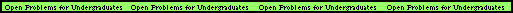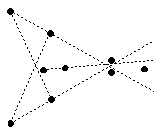# Combinatorial Geometry Open Problems

Index of Problems

### Crossing FamiliesRESEARCHER: Pavel Valtr
OFFICE: CoRE 411
Email:valtr@dimacs.rutgers.edu
DESCRIPTION: Consider N points in the plane so that no three points lie on a line. Draw a line segment between each pair of vertices. The open problem is this: How large a family of mutually crossing line segments must there be? We say two line segments cross if they intersect on their interiors (as opposed to at their endpoints.) In the figure to the right, there is a family of 3 mutually crossing line segments, but not a family of 4.

It has been shown that there must always be a family of size sqrt(N/12), but it is believed that there must always be families of much larger size as well. So, what is the largest exponent t so that among N points in the plane, no three on a line, one can always find a crossing family of at least cN^t line segments, for some fixed constant c>0? If you can find such a t bigger than 1/2, then you will have made a substantial contribution.### Empty HexagonsRESEARCHER: Pavel Valtr
OFFICE: CoRE 411
Email:valtr@dimacs.rutgers.edu
DESCRIPTION: It is known that any set of at least 10 points in the plane, no three on a line, contains an empty pentagon (i.e., 5 points which are vertices of a convex pentagon whose interior contains none of the points). The figure on the right shows 9 points with no empty pentagon (the dotted lines are shown to help visualization). Horton (1983) constructed arbitrarily large sets of points in the plane with no "empty 7-gon". The following question of Erdos remains open: "Is there an integer N such that any set of at least N points in the plane, no three on a line, contains an empty hexagon (i.e., six vertices of a convex hexagon whose interior contains no point of the set)?" Could you at least find, for any sufficiently large integer K, an empty hexagon in any set of K^2 points in a square K by K, when the distance between any two points is at least 1/10, say. This could be easier, although there is an example of such restricted sets with no empty heptagon.### Non-empty Strips

RESEARCHER: Pavel Valtr
OFFICE: CoRE 411
Email:valtr@dimacs.rutgers.edu
DESCRIPTION: Consider a set S of N^2 points inside a disk bounded by a circle C of length N, such that the distance between any two points of S is at least 1/100, say. Let A be a set of N^2 points distributed equidistantly along C. It is known that for at least c·N^4 pairs a,b of points of A, there is a point of S at distance at most 1/N from the line ab (c>0 is a positive constant). Is there a positive constant d>0 such that for at least d·N^4 pairs a,b of points of A, there are at least two points of S at distance at most 1/N from the line ab?Back to main page